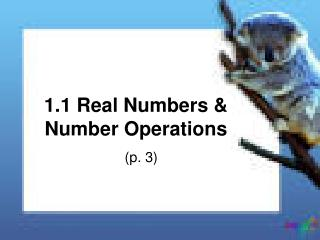DownloadDownload Presentation1.1 Real Numbers & Number Operations

# 1.1 Real Numbers & Number Operations

Download Presentation## 1.1 Real Numbers & Number Operations

- - - - - - - - - - - - - - - - - - - - - - - - - - - E N D - - - - - - - - - - - - - - - - - - - - - - - - - - -
##### Presentation Transcript

1. What is a real number? • All the numbers you are used to using in your previous math classes. • There are 4 types of real numbers: • Whole numbers • Integers • Irrational numbers • Rational numbers

2. Examples of Real numbers • Whole numbers: 0, 1, 2, 3 (counting #s) • Integers: -2, -1, 0, 1, 2 (+ & - whole #s) • Rational numbers: a # that can be written as a fraction. When written as a decimal, they terminate or repeat. ½, 1/3, 4/5, 7/9 • Irrational numbers: real #s that are not rational such as π or √3. Decimals that do not terminate or repeat.

3. Properties of Multiplication & Addition (a, b, & c are real #s) AdditionMultiplication Closure a+b is real a*b is real Commutative a+b=b+a ab=ba Associative (a+b)+c=a+(b+c) (ab)c=a(bc) Identity a+0=a, 0+a=a a*1=a, 1*a=a Inverse a+(-a)=0 a*(1/a)=1, a≠0 Distributive a(b+c)=ab+ac (a+b)c=ac+bc

4. Additive Inverse (opposite) ex: a and –a or -5 and 5 Multiplicative Inverse(reciprocal) ex: a and 1/a or -1/3 and -3 • Remember: • Difference means subtract • Quotient means divide

5. Unit Analysis Examples • 685ft + 225ft = 910ft • (2.25h) = 135km 3. 45 mi/h

6. Base ? Exponent ? Power ?

7. Ex: Evaluate the power • (-2)6 = (-2)*(-2)*(-2)*(-2)*(-2)*(-2) = 64 2. -26= -(2*2*2*2*2*2) = -64

8. Order of OperationsKnow these!! • Please Excuse My Dear Aunt Sally ( ) exponents multiply/divide (L to R) add/subtract (L to R)

9. Define: • Variable • Algebraic expression • Coefficient • Like terms • Constant term

10. Ex: Simplify the expression. • 6m2 – 12m – 7m2 = -m2 – 12m • 3(x-2) – 5(x-8) = 3x – 6 – 5x +40 = -2x + 34

11. Last example! • You want to buy either scented lotion or bath soap for 8 people. The lotions are \$6 each and the soaps are \$5 each. Using l for the # of lotions, write an expression for the total amount you must spend in terms of l. 6l + 5(8-l) 6l+40-5l l +40

12. Evaluate the expression when 5 people get lotion. l + 40 5 + 40 = 45 \$45

13. Assignment